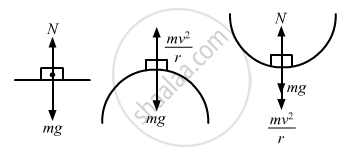Advertisement Remove all ads

# Three Identical Cars A, B and C Are Moving at the Same Speed on Three Bridges. the Car a Goes on a Place Bridge, B on a Bridge Convex Upward and C Goes on a Bridge Concave Upward. - Physics

MCQ

Three identical cars A, B and C are moving at the same speed on three bridges. The car A goes on a place bridge, B on a bridge convex upward and C goes on a bridge concave upward. Let FA, FB and FC be the normal forces exerted by the car on the bridges when they are at the middle of bridges.

#### Options

• FA is maximum of the three forces.

• FB is maximum of the three forces.

• FC is maximum of the three forces.

• FA = FB = FC

Advertisement Remove all ads

#### Solution

FC is maximum of the three forces.

At the middle of bridge, normal force can be given as : $\text{N}_\text{A} = \text{mg}$

$\text{N}_\text{B} = \frac{\text{mv}^2}{\text{r}} - \text{mg}$

$\text{N}_\text{C} = \frac{\text{mv}^2}{\text{r}} + \text{mg}$

So, FC is maximum.Is there an error in this question or solution?
Advertisement Remove all ads

#### APPEARS IN

HC Verma Class 11, 12 Concepts of Physics 1
Chapter 7 Circular Motion
MCQ | Q 11 | Page 113
Advertisement Remove all ads
Advertisement Remove all ads
Share
Notifications

View all notifications

Forgot password?